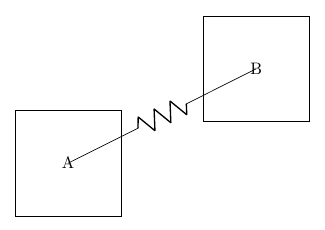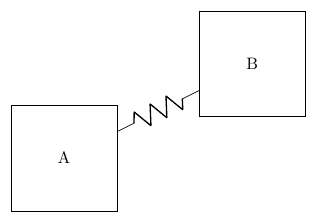# circuitikz wires overlapping nodes

Note: It turns out that this is an issue with circuitikz 1.0 and is apparently not a problem in the current version. A workaround would still be helpful, but it means the question isn't very urgent - I'll find a way to work around it for now and then update later.

I'm trying to draw some diagrams that mix circuit symbols with other notation. To do this I'm attempting to use circuitikz. However, it seems that when I attempt to add a circuit symbol to a path, it causes the path to overlap the nodes it's drawn between. Here's a minimal example - the only thing different between these paths is the use of [R] to draw a resistor.Is there a way I can prevent this from happening, so that the second diagram will look like the first, except with a resistor drawn in the middle of the line?

I know that I could fill the nodes and draw them after the line, and will do if I have to. However, in my actual document this is drawn with a series of macros and I'd have to change a lot to accomplish that. So I'm looking for an easier solution, if one exists.

Because it's drawn with macros, the solution needs to be automatic - it will be a lot of work for me if I manually need to specify things like A.east and B.west. In addition, the nodes aren't necessarily all directly above/below or left/right of each other in the real diagram.

Here's the mwe code:

\documentclass{article}
\usepackage{circuitikz}

% make the resistor a suitable size
\ctikzset{bipoles/resistor/height=0.1}
\ctikzset{bipoles/resistor/width=0.3}

\begin{document}

% two squares with a line between them
\begin{tikzpicture}
\node at (0,0) [draw] (A) {};
\node at (1,0) [draw] (B) {};
\draw (A) to (B);
\end{tikzpicture}

% the same but with a resistor drawn on the line
\begin{tikzpicture}
\node at (0,0) [draw] (A) {};
\node at (1,0) [draw] (B) {};
\draw (A) to[R] (B);
\end{tikzpicture}

\end{document}

• Try \draw (A.east) to[R] (B.west). Oct 2, 2020 at 1:18
• @nidhin that works for this simple example, but unfortunately my actual diagram is drawn with a series of macros, and in my case there's no way for the macros to know whether they should connect to the east or west or some other non-ordinal direction. I've edited the question to clarify that. Oct 2, 2020 at 1:20
• With recent circuitikz (version 1.2.3 (2020/08/07) I can't reproduce your result. As line as resistors start and end at nodes' bodes borders. Check if you have the recent version of circuitikz. Oct 2, 2020 at 2:10
• @Zarko \pgfcircversion displays "1.0", so I guess my version is out of date. I don't really want to mess with my TeX installation at the moment though, due to an upcoming deadline. So I will edit to specify that I need a solution for 1.0. Oct 2, 2020 at 2:39
• @Zarko still, that's helpful - it gives me an excuse to just hack some macros to work around it by drawing the nodes twice. Once the deadline's passed I'll update and won't have to do that any more. Oct 2, 2020 at 2:42

I suspect that the only practical solution is to upgrade circuitikz (which is really easy, even in a local form).

The problem was that from some archeological version (don't know when, really, it was before git times) the code to generate paths in circuitikz was changed so that if the path started at a node the starting point was forced to the coordinate node.center. (The code was made better by other developers, as you can see following the links, but basically retaining that behavior).

My personal opinion is that this was ill-advised (it fixed a couple of circuits that where wrong, using nodes instead of coordinates; but at that times I was not involved in the development and surely did not have the skills to be there, so...). That forced a series of workarounds in the code that became impossible to maintain in my opinion and led to problems at line junctions.

So I took the plunge and removed hacks and that change before 1.2.0. Your example is practically the same I used to defend the change, look here: https://github.com/circuitikz/circuitikz/issues/76#issuecomment-652980687:

To explain point 1 here: this code

    \node [draw](A) at (0,0) {A};
\node [draw](B) at (4,2) {B};
\draw (A) to[R] (B); \end{tikzpicture} 

renders asin 1.1.2 and previous and asnow --- you can easily have the old behavior (if you really wanted it) with using A.center and B.center explicitly, but it's really complex to obtain the new one --- which in the rest of tikz` is considered the correct one.